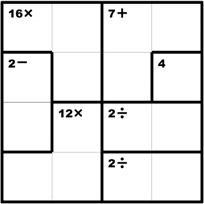### KenKen and Recursive Backtracking

For this assignment, you are to design and implement a Java program for solving KenKen puzzles. If you are not familiar with KenKen, it is played on an NxN puzzle grid in which the numbers 1 through N are placed.To complete a puzzle, the player must fill in the grid such that the numbers 1 through N appear in every row and column. Furthermore, sets of outlined squares (called cages) have mathematical constraints. For example, the top left square in the above puzzle is in a cage with the constraint "16x," which means that the three numbers in the cage have a product of 16 (i.e., 4*2*2 or 4*4*1).

For a person, solving a KenKen puzzle requires complex and careful logical reasoning. However, it turns out that even the most challenging KenKen puzzles can be easily solved by a computer using recursive backtracking. In pseudocode:

For each spot in the grid place a number in it if a conflict occurs (i.e., a duplicate in any row/column or a violated constraint), then remove the number and backtrack to try the next number if there is no conflict, attempt to (recursively) fill in the rest of the grid if you are unable to fill the rest of the grid (i.e., a future dead-end is reached), then remove the number and backtrack to try the next number

Your program should prompt the user for a file that contains the specifications for a puzzle. The first line of the puzzle file should specify the size of the puzzle (you may assume a maximum size of 9x9). Each subsequent line should identify a cage, with the constraint first (e.g., "16*") followed by the coordinates in the cage. For example, the above puzzle would be represented as:

4 16* (0,0) (0,1) (1,1) 7+ (0,2) (0,3) (1,2) 2- (1,0) (2,0) 4 (1,3) 12* (2,1) (3,0) (3,1) 2/ (2,2) (2,3) 2/ (3,2) (3,3)

Note that we will use the '*' and '/' characters to represent multiplication and division. You may assume that the formula and the coordinates on a line are separated by whitespace, but that no spaces appear within a formula or coordinate. Your program should display the solved puzzle if a solution exists, or display a message if no solution is possible. The solution to the above puzzle would be:

2 4 1 3 1 2 3 4 3 1 4 2 4 3 2 1